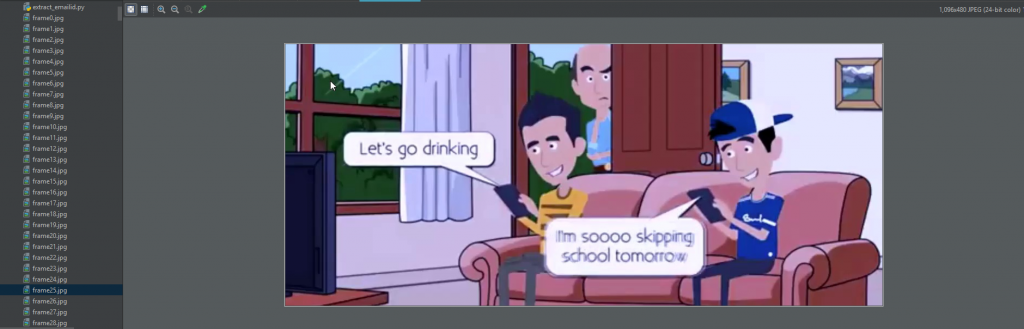GeeksforGeeks App
Open AppBrowser
Continue

# Python | Play a video in reverse mode using OpenCV

OpenCV (Open Source Computer Vision) is a computer vision library that contains various functions to perform operations on Images or videos.

```OpenCV's application areas include :

1) Facial recognition system
2) motion tracking
3) Artificial neural network
4) Deep neural network
5) video streaming  etc.```

In Python, one can use an OpenCV library named CV2. Python does not come with cv2, so user needs to install it separately.

For Windows :

`pip install opencv-python`

For Linux :

`sudo apt-get install python-opencv`

OpenCv library can be used to perform multiple operations on videos. Let’s try to do something interesting using CV2. Take a video as input and play it in a reverse mode by breaking the video into frame by frame and simultaneously store that frame in the list. After getting list of frames we perform iteration over the frames. For playing video in reverse mode, we need only to iterate reverse in the list of frames. Use reverse method of the list for reversing the order of frames in the list.
Below is the implementation :

## Python3

 `# Python program to play a video``# in reverse mode using opencv `` ` `# import cv2 library``import` `cv2`` ` `# videoCapture method of cv2 return video object`` ` `# Pass absolute address of video file ``cap ``=` `cv2.VideoCapture(``"video_file_location"``)`` ` `# read method of video object will return``# a tuple with 1st element denotes whether``# the frame was read successfully or not,``# 2nd element is the actual frame.`` ` `# Grab the current frame.``check , vid ``=` `cap.read()`` ` `# counter variable for``# counting frames``counter ``=` `0`` ` `# Initialize the value``# of check variable``check ``=` `True`` ` `frame_list ``=` `[]`` ` `# If reached the end of the video ``# then we got False value of check.`` ` `# keep looping until we``# got False value of check.``while``(check ``=``=` `True``):``     ` `    ``# imwrite method of cv2 saves the ``    ``# image to the specified format.``    ``cv2.imwrite(``"frame%d.jpg"` `%``counter , vid)``    ``check , vid ``=` `cap.read()``     ` `    ``# Add each frame in the list by``    ``# using append method of the List``    ``frame_list.append(vid)``     ` `    ``# increment the counter by 1``    ``counter ``+``=` `1`` ` `# last value in the frame_list is None``# because when video reaches to the end``# then false value store in check variable``# and None value is store in vide variable.`` ` `# removing the last value from the ``# frame_list by using pop method of List``frame_list.pop()`` ` `# looping in the List of frames.``for` `frame ``in` `frame_list:``     ` `    ``# show the frame.``    ``cv2.imshow(``"Frame"` `, frame)``     ` `    ``# waitkey method to stopping the frame``    ``# for some time. q key is presses,``    ``# stop the loop``    ``if` `cv2.waitKey(``25``) ``and` `0xFF` `=``=` `ord``(``"q"``):``        ``break``     ` `# release method of video ``# object clean the input video``cap.release()`` ` `# close any open windows``cv2.destroyAllWindows()`` ` `# reverse the order of the element ``# present in the list by using``# reverse method of the List.``frame_list.reverse()`` ` `for` `frame ``in` `frame_list:``    ``cv2.imshow(``"Frame"` `, frame)``    ``if` `cv2.waitKey(``25``) ``and` `0xFF` `=``=` `ord``(``"q"``):``        ``break`` ` `cap.release()``cv2.destroyAllWindows()`

Output :My Personal Notes arrow_drop_up